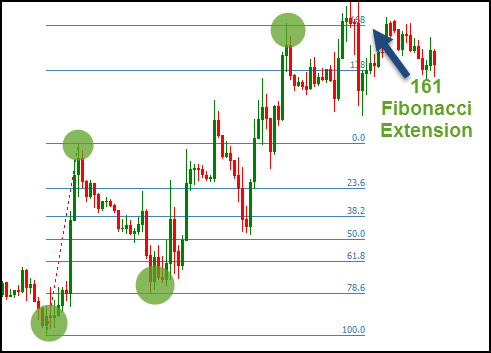--120 views

# Fibonacci: Price ExtensionFibonacci Extension Price or Fibonacci price extension is similar to Fibonacci Retracement. This is also a support and resistance indicator used to determine possible support and resistance levels.

Like Fibonacci Fans and Fibonacci Retracements, Fibonacci Extension is based on a mathematical relationship, expressed as the ratio, between numbers in the Fibonacci sum sequence. But unlike Fibonacci Retracement, Fibonacci Extension looks for support and resistance levels that may be more than 100% of the previous price movement.

The most important Fibonacci Extension levels can be found by performing various mathematical function operations on numbers in the Fibonacci sum sequence, and on the results of those operations.

The first ratio of 161.8%, which is the “golden ratio” or “golden average”, is found by dividing the number in sequence by the number that preceded it. For example: 21 ÷ 13 = 1.6154, 34 ÷ 21 = 1.6190 and 55 ÷ 34 = 1.6176.
The 261.8% ratio is found by dividing the numbers in a sequence with the numbers that appear two previous places. For example: 34 ÷ 13 = 2.425, 55 ÷ 21 = 2.619 and 144 ÷ 55 = 2.61818. This is also a box of 1,618 (1.618 x 1.618 = 2.618).
The ratio of 423.6% is found by dividing the numbers in a sequence with the numbers appearing three previous places. For example: 55 ÷ 13 = 4.2308, 89 ÷ 21 = 4.2381 and 144 ÷ 34 = 4.2353. This is also the sum of 1,618 and 2,618 (1,618 + 2,618 = 4,236).
In addition to these three ratios, an extension level of 127.2% is also used. This level is the square root of 1,618 (√1.618 = 1.272).
Fibonacci Extension is used in the same way as Fibonacci Retracement but with different directions. Technical analysts identify trends, then wait for the market to turn against the trend and then wait for the market to start moving back towards the dominant trend. The analyst then applies the extension ratio to the previous countertrend movement, or wave, starting from the previous low level to the high level in the uptrend and vice versa in the descending trend. The horizontal line is then drawn at this level and a possible resistance level is used if the dominant trend is the uptrend, or maybe the support level if the dominant trend is the descending trend.

These levels also serve as price targets when trading into the dominant trend. The most significant level is usually the 161.8% level followed by the 127.2% level. Level 261.8% and 423.6% level are also significant in the market which has a very strong trend movement.